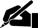# Term Paper: Calculate the Mean, Median, and Mode

Pages: 1 (312 words)  ·  Style: APA  ·  Bibliography Sources: 2  ·  Topic: Business - Marketing  ·  Buy for \$19.77

Calculate the mean, median, and mode for the data.

Mean = Sum of X values / N (Number of values)

Mean = 885 /

Mean = 68.0769

Median = 62 found by arranging the numbers in ascending order and selecting the value in the (n + 1) / 2 position which is the 7th position or

56, 56, 62, 62, 62, 62, 62, 70, 75, 75, 75, 81, 87

Mode = 62 found by selecting the most frequently occurring value

Prepare a frequency distribution for the data.

Rank

Occurrences

Calculate the standard deviation for this data.

Standard Deviation = sqrt (sum (value - mean) 2) / N)

Standard Deviation = 9.78487

Explain why statisticians typically use the standard deviation rather than the average deviation.

The standard deviation is a statistic that tells you how tightly all the various examples are clustered around the mean in a set of data (Niles). When the examples are pretty tightly… [END OF PREVIEW]

### Ordering Options:

or

2.  Let us write a NEW paper for you!Most popular!

#### Articles Published on Criminal Justice and Restorative Term Paper…

Cite This Term Paper:

APA Format

Calculate the Mean, Median, and Mode.  (2008, March 14).  Retrieved November 17, 2019, from https://www.essaytown.com/subjects/paper/calculate-mean-median-mode/4264

MLA Format

"Calculate the Mean, Median, and Mode."  14 March 2008.  Web.  17 November 2019. <https://www.essaytown.com/subjects/paper/calculate-mean-median-mode/4264>.

Chicago Format

"Calculate the Mean, Median, and Mode."  Essaytown.com.  March 14, 2008.  Accessed November 17, 2019.
https://www.essaytown.com/subjects/paper/calculate-mean-median-mode/4264.